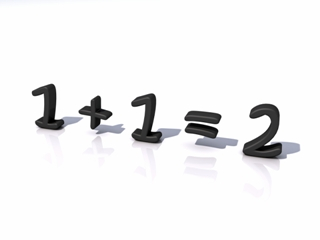# Cool equation

Algebra Level 3How many ordered solutions $(x ,y)$ are there for the equation:

${2}^{{x}^{2}} + {2}^{{y}^{2}} = 1 + {cos}^{2} x$

×

Problem Loading...

Note Loading...

Set Loading...